# The A* Algorithm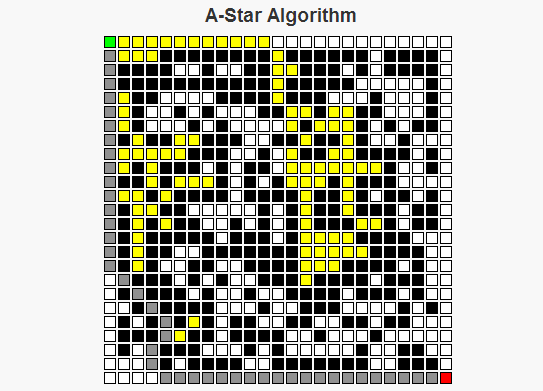As a child I remember traveling on road trips, sitting in the back of a car trying to do my best to keep myself busy for what would occupy the next six to ten hours of my life. One of the things I grew to like were the simple maze books that were sold on the magazine racks at some of the gas stations where we’d stop for food. There are two basic strategies I employed for solving these mazes: For simpler mazes, I could generally just take a look at the overall maze structure, decide upon a path through the maze, then write a path without any mistakes. For more complex mazes though, I would generally begin a route that looks the most promising. If that route reaches a point where I can see that it will be impossible to finish, then I’d go back to where the decision was made, exclude the route I had just tried, and select the “most promising” remaining route. I’d continue this process until I had completed the maze, or until it became simple enough for me to solve the maze using only my memory.

The A* Algorithm works in a similar manner to the second approach I just described. We begin at a starting point, and consider where to move next from that starting point. The set of possible options for this next move is determined by the neighbor function for a given cell. For each neighbor the algorithm estimates the length of the route through that cell by calculating the sum of two values, f(n) = g(n) + h(n), where

g(n) is the (known) cost to travel from the starting point to the cell n.
h(n) is the (approximate) cost to travel from the cell n to the final cell.

The sum g(n) + h(n) allows us to approximate the total cost of a route through the cell n.

The A* algorithm begins at the starting position of the maze. There are two sets we will be considering throughout the process of determining the optimal route, called the closedSet and the openSet. The elements of closedSet are the nodes whose total distance from the starting position has been calculated, whereas the elements of openSet represents nodes whose total distance is still under consideration. There is also a map called prev which is used to reconstruct the path. Below is how the algorithm operates:

A* Algorithm Pseudocode
closedSet is the empty set
openSet = {start}
prev is the empty set

While there are still elements in openSet,
Find the element c* in openSet with the lowest value f(c).
If c* is the target position
Reconstruct the path.
Else If c* is not the target position,
Remove c* from openSet
For each neighbor n of c* that is not in closedSet,
Calculate a temporary g value, temp_g(n) = g(c*) + dist(c*, n).
If n is not in openSet, or if n is in openSet and temp_g(n) < g(n),                     Set prev(n) = c*                     Set g(n) = temp_g(n)                     Set f(n) = g(n) + h(n).                     if n is not in openSet                          add n to openSet.      End If End While End AlgorithmAn important question becomes what makes a good heuristic function to approximate the distance to the goal. This can lead to an in depth discussion based on the word "good", but the necessary condition for any heuristic is that it NEVER over-estimates the cost of the path from the cell to the goal. Some examples of possible heuristics for mazes are the Euclidean Distance (the square root of the sum of the squares of the horizontal and vertical differences in distances, i.e. dE(x, y) =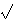(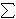i(xi – yi)2) and TaxiCab Distance (the sum of the differences in the horizontal and vertical dimensions, i.e. dT(x, y) =i|xi – yi|). Both of these are feasible metrics for heuristics on a maze. Other herusitics, like h(n) = 0 for all n are possible, but doing this would make the algorithm treat all cells equally and ignore the heuristic part of the A* algorithm, turning it into Dijkstra’s algorithm.

I’ve written a script that generates random mazes and uses the A* algorithm to find the optimal path through this maze. For this script, I used the taxicab distance heuristic.

Check it out and let me know what you think.

# Simple Linear Regression

We live in a world that is filled with patterns – patterns all around us just waiting to be discovered. Some of these patterns are not as easily discovered because of the existence of outside noise.

Consider for example an experiment where a set of people were each given the task of drinking a number of beers and having their blood alcohol level taken afterwards. Some noise factors in this could include the height and weight of the individual, the types of drinks, the amount of food eaten, and the time between drinks. Even with this noise, though, we can still see a correlation between the number of drinks and their blood alcohol level. Consider the following graph showing people’s blood-alcohol level after a given number of drinks. The x-axis represents the number of drinks and the y-axis is the corresponding blood alcohol level.x 5 2 9 8 3 7 3 5 3 5 4 6 5 7 1 4 y 0.1 0.03 0.19 0.12 0.04 0.095 0.07 0.06 0.02 0.05 0.07 0.1 0.085 0.09 0.01 0.05

We can definitely see a correlation, and although the data doesn’t quite fit on a straight line. It leads us to ask further questions like can we use this data to build a model that estimates a person’s blood-alcohol level and how strong is this model?

One of the tools we can use to model this problem is linear regression. A linear regression takes a two-dimensional data set, with the assumption that one column (generally represented by the x variable) is independent and the second column (generally represented by the y variable) being dependent on the first column. The assumption is that the relationship between the two columns is linear and can be represented by the linear equation

y =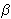0 +1x + e.

The right hand side of the above equation has three terms. The first two (0 and1) are the parameters of the linear equation (the y-intercept and slope respectively), while the third term of the right hand side of the above equation represents the error term. The error term represents the difference between this linear equation and the y values in the data provided. We are seeking a line that minimizes the error term. That is, we are seeking to minimize

D =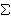i = 1 to n [yi – (0 +1xi)]2

There are several ways one could approach this problem. In fact, there are several lines that one could use to build a linear model. The first line that one may use to model these points is the one generated by only mean of the y values of the points, called the horizontal line regression.

For the data set above, the mean of the y values can be calculated as= 0.0738, so we could build a linear model based on this mean that would be y = 0.738. This horizontal line regression model is a horizontal line that predicts the same score (the mean), regardless of the x value. This lack of adjustments means it is generally a poor fit for most models. But as we will see later, this horizontal line regression model does serve a purpose in determining how well the model we develop performs.

A second attempt at solving this problem would be to generate the least squares line. This is the line that minimizes the D value listed above. We can see that D is a multi-variable polynomial, and we can find the minimum of such a polynomial using calculus, partial derivatives and Gaussian elimination (I will omit the work here because it deters us from the main point of this blog post, however Steven J. Miller has a good write-up of this).

The calculus leads us to the following equations:

SXY =i = 1 to n(xy) –
 (i = 1 to nx)(i = 1 to ny) n
SXX =i = 1 to n(x2) –
 (i = 1 to nx)2 n1 =
 SXY SXX0 =–1To calculate the least squares line for this example, we first need to calculate a few values:i = 1 to n(xy) = 6.98i = 1 to n(x2) = 443i = 1 to nx = 77i = 1 to ny = 1.18
Sxx = 72.44
Sxy = 1.30

This lets us evaluate that1 = 0.018
and0 = -0.0127

So the resulting linear equation for this data is= -0.0127 + 0.018*x

Below is a graph of the two attempts at building a linear model for this data.In the above image, the green line represents the horizontal line regression model and the blue line represents the least-squares line. As stated above, the horizontal line regression model is a horizontal line that does not adjust as the data changes. The least-squares line adjusts both the slope and y-intercept of this line according to the data provided to better fit the data provided. The question becomes how well does the least-squares line fit the data.

The Sum of Squares Error (SSE) sums the deviation at each point of our data from the least-squares line.

SSE =i = 1 to n(yii)2

A second metric that we are interested in is how well the horizontal line regression linear model estimates our data. This is called the Total Sum of Squares (SST).

SST =i = 1 to n(yi)2

The horizontal line regression model ignores the independent variable x from our data set and thus any line that takes this independent variable into account will be an improvement on the horizontal line regression model. Because of this, the SST sum is a worse case scenario of how poorly our model can perform.

Knowing now that SST is always greater than SSE, the regression sum of squares (SSR) is the difference between the total sum of squares and the sum of squares error.

SSR = SST – SSE

This tells us how much of the total sum of squares is accounted for by the model.

Finally, the coefficient of determination (r2) is defined by

r2 = SSR / SST

This tells SSR as a percentage of SST, or the amount of the variation in the data that is explained by the model.

So, check out my script on simple linear regression and let me know what you think.